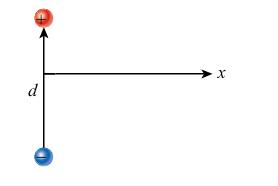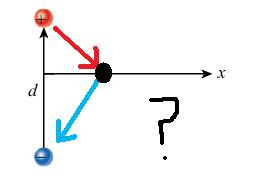# Electric Dipole Electric Field

Electric Dipole Electric Field.. URGENT

## Homework Statement

For the electric dipole shown in the figure, express the magnitude of the resulting electric field as a function of the perpendicular distance x from the center of the dipole axis in terms of the electric dipole moment, p.E = Fq
P = qd
E = kq/(r^2)

## The Attempt at a Solution

R = sqrt[(d/2)^2 + x^2]
q = P/d

Thus,

E = kP/{d*[sqrt(d/2)^2+x^2]^2}

Since I'm looking for the x-component, multiply E by the cos(theta), which in this case is x/R, or...

cos(theta) = x/sqrt[(d/2)^2+x^2]

so the answer i get is....

E = kP/{d*[sqrt(d/2)^2+x^2]^2} * x/sqrt[(d/2)^2+x^2]

which comes out to be...

E = kP/[sqrt(d/2)^2+x^2]^(3/2) * x/d

E = kP/[sqrt(d/2)^2+x^2]^(3/2)

So my question is, what happens to the x/d?

berkeman
Mentor

## Homework Statement

For the electric dipole shown in the figure, express the magnitude of the resulting electric field as a function of the perpendicular distance x from the center of the dipole axis in terms of the electric dipole moment, p.E = Fq
P = qd
E = kq/(r^2)

## The Attempt at a Solution

R = sqrt[(d/2)^2 + x^2]
q = P/d

Thus,

E = kP/{d*[sqrt(d/2)^2+x^2]^2}

Since I'm looking for the x-component, multiply E by the cos(theta), which in this case is x/R, or...

cos(theta) = x/sqrt[(d/2)^2+x^2]

so the answer i get is....

E = kP/{d*[sqrt(d/2)^2+x^2]^2} * x/sqrt[(d/2)^2+x^2]

which comes out to be...

E = kP/[sqrt(d/2)^2+x^2]^(3/2) * x/d

E = kP/[sqrt(d/2)^2+x^2]^(3/2)

So my question is, what happens to the x/d?

You are not "looking for the x-component" (Quiz Question -- why not?)

You are looking for the net electric field as a function of the distance out on the x-axis. What direction is the resultant E field pointing?

The resultant E field would be a curved path, clockwise from the + to the - charge.

We're looking for the E field as a function of the perpendicular distance, but what does that mean if it's not the x-component?

And then, how do you go about solving for it?

berkeman
Mentor

The resultant E field would be a curved path, clockwise from the + to the - charge.

We're looking for the E field as a function of the perpendicular distance, but what does that mean if it's not the x-component?

And then, how do you go about solving for it?

They ask only for the E-field on the x-axis. That means you will solve for E(x). You do that by going to a point x on the x-axis, and adding up the two E-field vectors from the two point charges. Some of those vector components will cancel when they are added, and others will give a non-zero resultant.

On the figure shown, go to a point out on the x-axis to the right, and draw the two E-field vectors for the two point charges (only at that point). What would you get when you add those two vectors?

This is what I've come up with.but now I'm completely lost.

This is what else I've come up with...

EXred = red*cos(theta) --theta being the angle between the red vector and x-axis
EYred = red*sin(theta)

EXblue = blue*cos(theta) --angle between the blue vector and the x-axis
EYblue = blue*sin(theta)

so you add the X and Y directions, the X cancels out, and youre left with only Y?

berkeman
Mentor

This is what else I've come up with...

EXred = red*cos(theta) --theta being the angle between the red vector and x-axis
EYred = red*sin(theta)

EXblue = blue*cos(theta) --angle between the blue vector and the x-axis
EYblue = blue*sin(theta)

so you add the X and Y directions, the X cancels out, and youre left with only Y?

Very good! Now use the formula for E-field as a function of distance (the distance to the point x for each is the hypoteneus of each triangle, right?), and add up the y-components. You're almost there. Be sure to express the sum as a vector, saying which direction in y the resultant points.